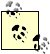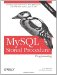# Recursion

A recursive routine is one that invokes itself. Recursive routines often offer elegant solutions to complex programming problems, but they also tend to consume large amounts of memory. They are also likely to be less efficient and less scalable than implementations based on iterative execution.

Most recursive algorithms can be reformulated using nonrecursive techniques involving iteration. Where possible, we should give preference to the more efficient iterative algorithm.

For example, in Example 22-18, the stored procedure uses recursion to calculate the Nth element of the Fibonacci sequence, in which each element in the sequence is the sum of the previous two numbers.

Example 22-18. Recursive implementation of the Fibonacci algorithm

 ```CREATE PROCEDURE rec_fib(n INT,OUT out_fib INT) BEGIN DECLARE n_1 INT; DECLARE n_2 INT; IF (n=0) THEN SET out_fib=0; ELSEIF (n=1) then SET out_fib=1; ELSE CALL rec_fib(n-1,n_1); CALL rec_fib(n-2,n_2); SET out_fib=(n_1 + n_2); END IF; END ```

Example 22-19 shows a nonrecursive implementation that returns the Nth element of the Fibonacci sequence.

Example 22-19. Nonrecursive implementation of the Fibonacci sequence

 ```CREATE PROCEDURE nonrec_fib(n INT,OUT out_fib INT) BEGIN DECLARE m INT default 0; DECLARE k INT DEFAULT 1; DECLARE i INT; DECLARE tmp INT; SET m=0; SET k=1; SET i=1; WHILE (i<=n) DO SET tmp=m+k; SET m=k; SET k=tmp; SET i=i+1; END WHILE; SET out_fib=m; END ```

Figure 22-9 compares the relative performance of the recursive and nonrecursive implementations. Not only is the recursive algorithm less efficient for almost any given input value, it also degrades rapidly as the number of recursions increases (which is, in turn, dependent on which element of the Fibonacci sequence is requested). As well as being inherently a less efficient algorithm, each recursion requires MySQL to create the context for a new stored program (or function) invocation. As a result, recursive algorithms tend to waste memory as well as being slower than their iterative alternatives.

Figure 22-9. Performance of recursive and nonrecursive calculations of Fibonacci numbers (note logarithmic scale)The maximum recursion depththe number of times a procedure can call itselfis controlled by the MySQL configuration parameter max_sp_recursion_depth. The default value of 0 disables all recursive procedures. A procedure that attempts to recurse beyond the value of max_sp_recursion_depth will encounter a runtime error:

``` mysql> CALL rec_fib(10,@x);
ERROR 1456 (HY000): Recursive limit 0 (as set by the max_sp_recursion_depth variable)
was exceeded for routine rec_fib
```

Recursion in stored functions is not allowed. An attempt to recurse in a function will always generate a runtime error:

``` mysql> SELECT rec_fib(10);
ERROR 1424 (HY000): Recursive stored functions and triggers are not allowed.
```Recursive solutions rarely perform as efficiently as their nonrecursive alternatives.MySQL Stored Procedure Programming
ISBN: 0596100892
EAN: 2147483647
Year: 2004
Pages: 208